(3)   The redshift

Suppose subscript  “1”  represent  “2”  represent the place of emitting photon and subscript “2”  represent the place of receiving photon. Thus redshift is defined as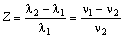Z>0  is the redshift,  Z<0  the blueshift.

1)  Velocity redshift

The velocity redshift is Doppler effect due to the velocity  V  between celestial bodies, we know that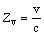(11)

and relativistic correction term of the  Zv  is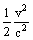2)  Repulsion redshift

Just as gravitation have an effect on light so repulsion have spectrum arise frequency shift. According to the law of conservation of energy we have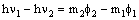here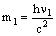,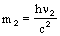,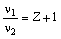we obtain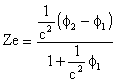(12)

3) Total redshift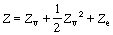(13)

4) Maximum apparent redshift

When  Zv=1  total redshift is

Zmax=1.5 + Ze

the maximum of redshift will have been determined by observation. If we can’t find a greater redshift than some value, then this value that is  Zmax. The last selection of cosmic parameter depend on  Zmax.

to agreat extent.